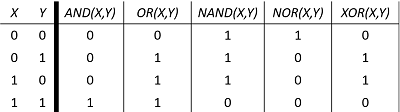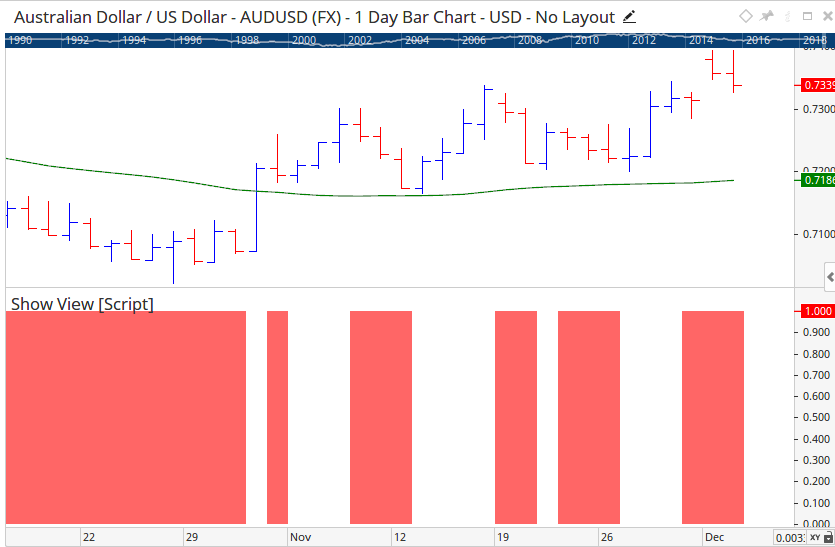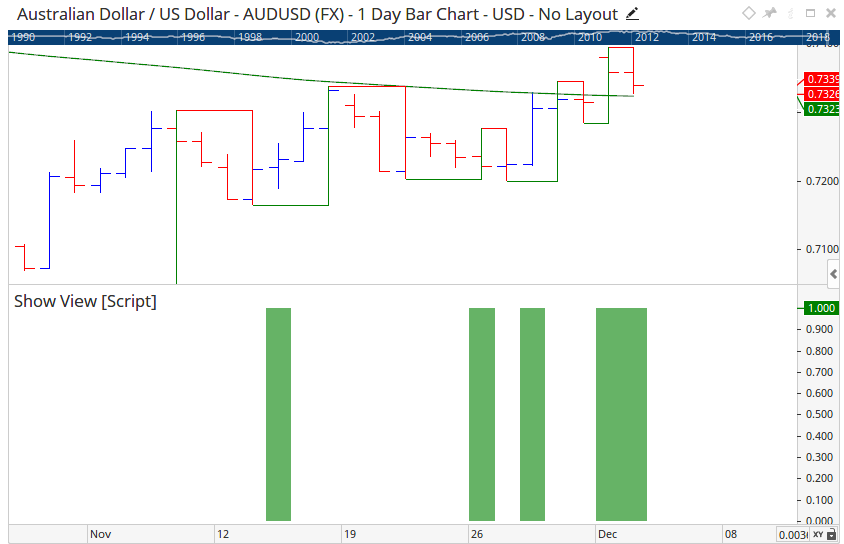# Logical Operators

Author: Optuma Team Last updated: Apr 3, 2020 05:22

## Overview

The following Logical Operators are available for use in the Optuma Scripting Language:

• AND
• OR
• NAND
• NOR
• XOR

The following table is a reference to how each Operator will function in scripting:## Examples

In the following script, both criteria (V1 and V2) must pass for a True (1) result to be returned.

``````V1 = CLOSE() &gt; OPEN() ;
V2 = CLOSE() &gt; MA(BARS=50) ;
V1 and V2
``````If we swapped the Logical Operator in the same script to NAND the results would invert and would only show a True result if only 1 or neither of the criteria passed.

``````V1 = CLOSE() &gt; OPEN() ;
V2 = CLOSE() &gt; MA(BARS=50) ;
V1 nand V2
``````In the following script either criteria (V1 or V2) must pass for a True (1) result to be returned.

``````V1 = GANNSWING() TURNSUP ;
V2 = CLOSE() > MA(BARS=150) ;
V1 or V2
``````If we swapped the Logical Operator of the same script to NOR the results would invert and only show a True result when both criteria did not pass.

``````V1 = GANNSWING() TURNSUP ;
V2 = CLOSE() &gt; MA(BARS=150) ;
V1 nor V2
``````In the following script only one of the two listed criteria (V1 or V2) can pass for a True (1) result to be returned. If both pass, or both fail the script will return a False (0) result.

``````V1 = MA(BARS=25) CrossesAbove MA(BARS=150) ;
V2 = CLOSE() &gt; MA(BARS=150) ;
V1 xor V2
``````